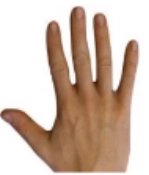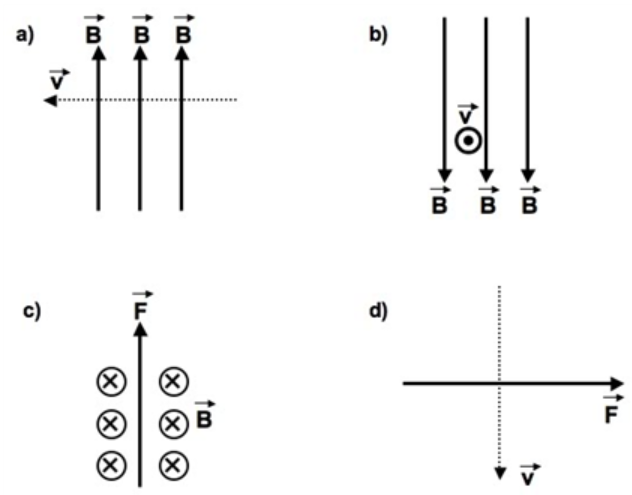# Problem: 1. In the arrangements shown, assume a positive electrical charge is moving withvelocity v through a magnetic field B.Answer the following questions about theindicated Figure:Figure (a): Show the direction of the forceFigure (b): Show the direction of the forceFigure (c): Show the direction of the velocityFigure (d): Show the direction of the magnetic field.2. Repeat each part of Question #1 for anegative charge.

###### FREE Expert Solution

In this problem, we're going to use the right-hand rule for positive charges and the left-hand rule for negative charges.

Put the right hand as shown:The four fingers point in the direction of the magnetic field.

The thumb points in the direction of velocity.

The palm points in the direction of the magnetic force.

We'll repeat the same for the negative charge, but using the left hand.

1. We use the right hand in this part since we're dealing with positive charges.

Figure (a)

Put the fingers in an upward direction and the thumb in the left direction.

93% (434 ratings)###### Problem Details1. In the arrangements shown, assume a positive electrical charge is moving with
velocity v through a magnetic field B.Answer the following questions about the
indicated Figure:
Figure (a): Show the direction of the force
Figure (b): Show the direction of the force
Figure (c): Show the direction of the velocity
Figure (d): Show the direction of the magnetic field.
2. Repeat each part of Question #1 for a
negative charge.